# DAV Class 5 Maths Chapter 10 Brain Teasers Solutions

The DAV Books Solutions Class 5 Maths and DAV Class 5 Maths Chapter 10 Brain Teasers Solutions of Averages offer comprehensive answers to textbook questions.

## DAV Class 5 Maths Ch 10 Brain Teasers Solutions

Question 1.
(a) The average of five numbers is 6. The sum of five numbers is _________
(i) 20
(ii) 10
(iii) 30
(iv) 35
Solution:
Average = $$\frac{Sum of numbers}{Total number of addends}$$
Given average = 6, no. of addends = 5
6 = $$\frac{Sum of numbers}{5}$$
Sum of numbers = 30
So option (iii).

(b) The average of the first five odd numbers is _________
(i) 5
(ii) 3
(iii) 7
(iv) 9
Solution:
First five odd numbers = 1,3, 5, 7, 9
Average = $$\frac{1+3+5+7+9}{5}$$
= $$\frac{25}{5}$$
So option (i)(c) The average of $$\frac{1}{4}$$ and $$\frac{3}{4}$$ is _________
(i) $$\frac{1}{2}$$
(ii) $$\frac{1}{4}$$
(iii) 1
(iv) $$\frac{3}{2}$$
Solution:
Average = $$\frac{\frac{1}{4}+\frac{3}{4}}{2}$$
= $$\frac{4}{4 \times 2}$$
= $$\frac{1}{2}$$
So option (i).

(d) The average of 4.8, 3.9, 8.6, 2.3 is _________
(i) 3.8
(ii) 4.9
(iii) 6.2
(iv) 5.9
Solution:
Average = $$\frac{4.8+3.9+8.6+2.3}{4}$$
= $$\frac{19.6}{4}$$
= 4.9
So option (ii).

(e) The average of three numbers is 6. If two of the numbers are 6 and 7, the third number is _________
(i) 5
(ii) 6
(iii) 4
(iv) 3
Solution:
Let the third number be x.
Average = 6, no. of addends = 3
Average = $$\frac{6+7+x}{3}$$
6 × 3 = 6 + 7 + x
18 = 13 + x
x = 18 – 13 = 5
So option (i).Question 2.
Find the average of the following sets of numbers:
(a) 60, 24, 126, 200, 185, 92
Solution: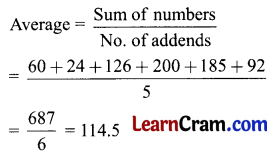(b) $$\frac{2}{10}, \frac{5}{10}, \frac{6}{10}, \frac{7}{10}, \frac{8}{10}$$
Solution:(c) 26.5, 15.4, 16.8, 20.9, 10.9
Solution: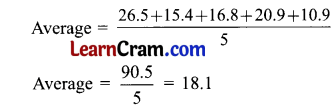Question 3.
Find the average of the first ten counting numbers.
Solution:
Average = $$\frac{1+2+3+4+5+6+7+8+9+10}{10}$$
= $$\frac{55}{10}$$
= 5.5Question 4.
Find the average of the first five multiples of 5.
Solution:
First five multiples = 5, 10, 15, 20, 25
Average = $$\frac{Sum of no.}{No. of addends}$$
= $$\frac{5+10+15+20+25}{5}$$
= $$\frac{75}{5}$$
= 15

Question 5.
The average age of three brothers is 15 years. If the age of the two brothers is 15 years and 12 years, find the age of the third brother.
Solution:
Let the age of the third brother = x yrs
Average age of three brothers = $$\frac{15+12+x}{3}$$
15 = $$\frac{27+x}{3}$$
15 × 3 = 27 + x
45 – 27 = x
x = 18 yrs
The age of the third brother is 18 years.

Question 6.
The heights of four members of a family are 1 m 60 cm, 1 m 50 cm, 1 m 10 cm, and 1 m 16 cm respectively. Find the average height of one member of the family.
Solution:
Average of one member of family = $$\frac{Sum of heights}{No. of members}$$
= $$\frac{1.60+1.50+1.10+1.16}{4}$$
= $$\frac{5.36}{4}$$
= 1.34 m
1.34 m is the average height of one member of the family.Question 7.
In a school, the number of girls and boys in three classes are given below:(a) Find the average number of girls in a class.
Solution:
Average number of girls in a class = $$\frac{Sum of no. of girl in a class}{Number of classes}$$
= $$\frac{24+26+28}{3}$$
= $$\frac{78}{3}$$
= 26
26 is the average number of girls in a class.

(b) Find the average number of boys in a class.
Solution:
Average number of boys in a class = $$\frac{Sum of no. of boys in a class}{Number of classes}$$
= $$\frac{34+38+39}{3}$$
= $$\frac{111}{3}$$
= 37
37 is the average no. of boys in a class.

(c) Find the total number of students in all three classes.
Solution:
Total number of students = No. of girls + No. of boys
= 78 + 111
= 189
189 is the total number of students in all three classes.

(d) What is the average number of students in a class?
Solution:
Average number of students in a class = $$\frac{189}{3}$$ = 63
63 students is the average number of students in a class.

Question 1.
Find the average of the following set of numbers:
(a) 3, 13, 20, 25, 8
Solution: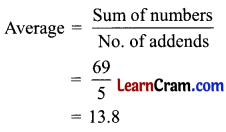(b) $$\frac{6}{20}, \frac{7}{20}, \frac{9}{20}, \frac{5}{20}, \frac{6}{20}$$
Solution: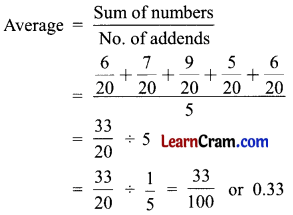(c) 20, 24, 25, 26, 27, 28
Solution: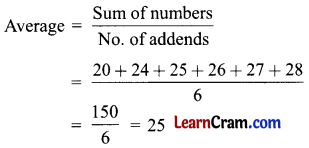Question 2.
Complete the table by finding the average of these sets.Solution:Question 3.
Find the average of the following:
(i) 3.2, 2.2, 2.6, 3.8, 2.8
Solution:
Average = $$\frac{3.2 + 2.2 + 2.6 + 3.8 + 2.8}{5}$$
= $$\frac{14.6}{5}$$
= 2.92

(ii) 0.5, 5.5, 2.5, 3.5, 4.5
Solution:
Average = $$\frac{0.5+5.5+2.5+3.5+4.5}{5}$$
= $$\frac{16.5}{5}$$
= 3.3

(iii) 1 m 50 cm, 2 m 30 cm, 4 m 20 cm, 3 m 25 cm, 5 m 20 cm
Solution:
Average = $$\frac{1.50+2.30+4.20+3.25+5.20}{5}$$
= $$\frac{16.45}{5}$$
= 3.29

Question 4.
Fill in the blanks:
(i) The average of 5, 15, and 10 is _________
Solution:
10

(ii) We divide _________ by _________ to find the average of 2, 3, 4.
Solution:
9, 3

(iii) We find the average by adding all the numbers and dividing the number of _________
Solution:Average = $$\frac{Sum of numbers}{Number of addends}$$
8 = $$\frac{7+9+x}{3}$$